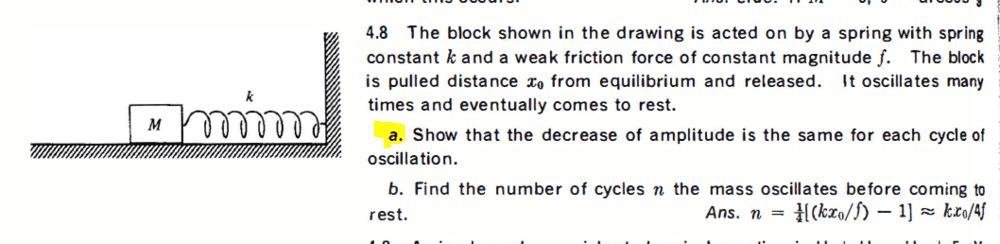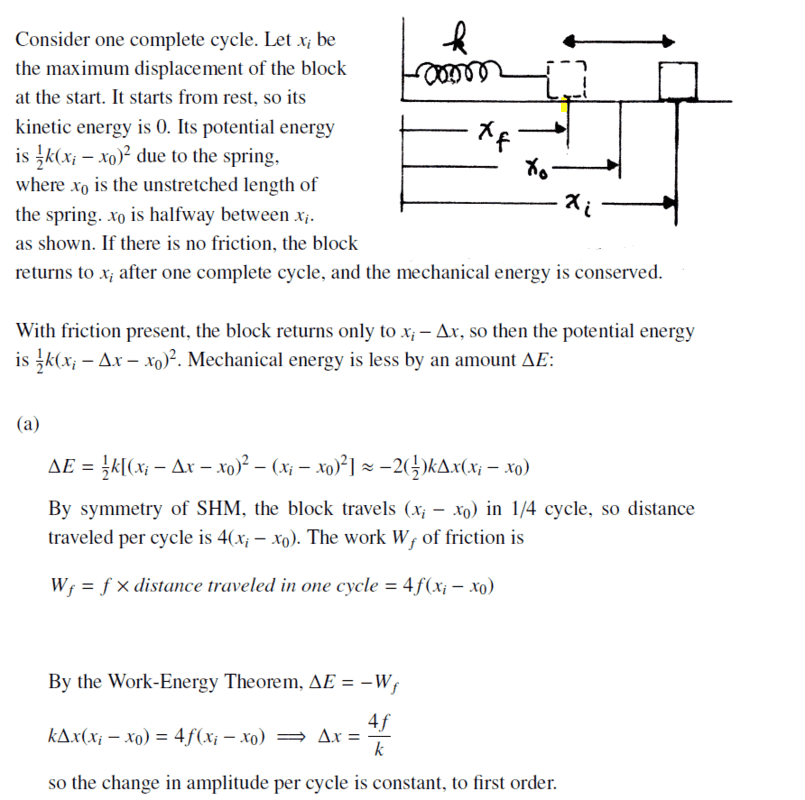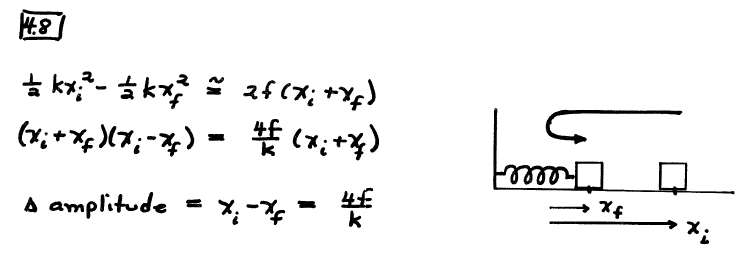# SHM retarded by friction

• Leo Liu

#### Leo Liu

Homework Statement
below
Relevant Equations
naSolution in 2ed manual:Solution in 1ed manual:Could someone explain why the work done by friction is multiplied by 2? I get that the distance traveled by the block in one cycle is greater than ##x_f+x_i##, but why is the coefficient two?

Homework Statement:: below
Relevant Equations:: na

View attachment 276734
Solution in 2ed manual:
View attachment 276735
Solution in 1ed manual:
View attachment 276736
Could someone explain why the work done by friction is multiplied by 2? I get that the distance traveled by the block in one cycle is greater than ##x_f+x_i##, but why is the coefficient two?
It is confusing that the two solutions use xi and xf to mean different things.
In the second one (1ed), they are both offsets from the relaxed position instead of from the wall, and xf means the new rightmost extension after one cycle.
That cycle involves traveling xi to the left to reach xo, then another (xi+xf)/2 to the left for its first leftmost position, then back through xo to its new rightmost position.
Total distance, 2(xi+xf).

•Lnewqban and Leo Liu